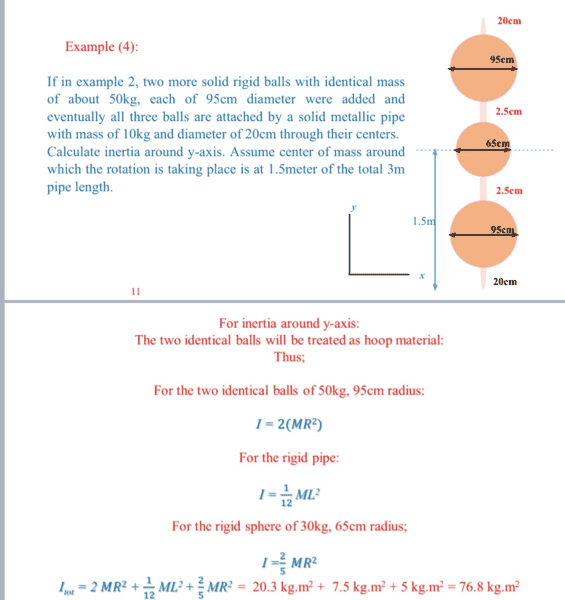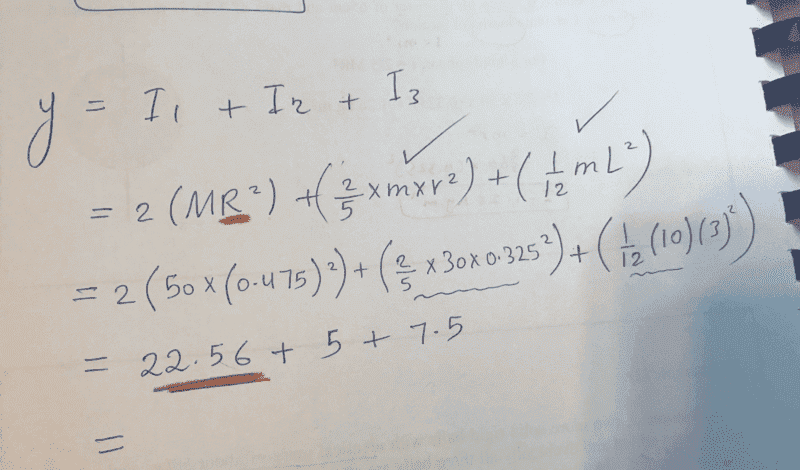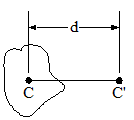# Rotational moment of inertia for 3 balls on a pipe

SakuRERE

## Homework Statement## The Attempt at a Solutioni just don't know from where to take the R which is colored with brown, in the 2(MR^2), what is the R here exactly, because i took the radius of the sphere , but i get different answer althought it's near.

#### Attachments

Homework Helper
Gold Member
Why is the moment of inertia of the rigid pipe about the y-axis ##(1/12)m L^2##? That's the moment of inertia about the x-axis passing through the mid point of the rod.

•SakuRERE
SakuRERE
Why is the moment of inertia of the rigid pipe about the y-axis ##(1/12)m L^2##? That's the moment of inertia about the x-axis passing through the mid point of the rod.
yeah the sysytem will be rotating around z ( perpendicular to the page) as we have (x,y) fixed point, so the system will be rotating in the plane of x and y but around z. so the axis of rotation z is perpendicular to the pipe and at the center of it, so that why i used 1/12.

Homework Helper
Gold Member
2022 Award
the sysytem will be rotating around z
Why do you think that?
The text says it is about the y axis. However, that wouid mean the information about the length of the rod and the spacing of the balls is irrelevant. I notice also the dashed horizontal line through the mass centre. All of this suggests the text is wrong and that the rotation is about the x axis.

Mentor
I am dubious of their assumption that the two identical balls can be "treated as hoop material". I think that will introduce a significant error (I estimate a bit more 10 %).
i just don't know from where to take the R which is colored with brown, in the 2(MR^2), what is the R here exactly, because i took the radius of the sphere , but i get different answer althought it's near.
I would think that it should be the distance of the centers of the large spheres from the axis of rotation.

Homework Helper
Gold Member
2022 Award
That's the moment of inertia about the x-axis passing through the mid point of the rod.
Not quite. The rod has a given diameter.

SakuRERE
Why do you think that?
The text says it is about the y axis. However, that wouid mean the information about the length of the rod and the spacing of the balls is irrelevant. I notice also the dashed horizontal line through the mass centre. All of this suggests the text is wrong and that the rotation is about the x axis.
you mean it will not move like a pinwheel?

SakuRERE
I am dubious of their assumption that the two identical balls can be "treated as hoop material". I think that will introduce a significant error (I estimate a bit more 10 %).

I would think that it should be the distance of the centers of the large spheres from the axis of rotation.
so the radius of the big sphere + 2.5+ the radius of the center sphere?

Mentor
so the radius of the big sphere + 2.5+ the radius of the center sphere?
That would be my view, yes.

Homework Helper
Gold Member
2022 Award
you mean it will not move like a pinwheel?
Because of the rotational symmetry about the y axis, it actually doesn't matter whether it is the x-axis or the z axis. I was just puzzled that you thought it was the z axis. As I posted, the text is probably wrong, so using x or z should be fine.

•gneill
SakuRERE
Because of the symmetry, it actually doesn't matter whether it is the x-axis or the z axis. I was just puzzled that you thought it was the z axis. As I posted, the text is probably wrong, so using x or z should be fine.
yeah i understood that the text says , it will rotate in the plane of x-y. but to make sure, it will move like a pin wheel right? and what about the R so? which R should i take?

Homework Helper
Gold Member
2022 Award
i get different answer althought it's near.

SakuRERE
so how am i supposed to solve it. i am so confused really

Homework Helper
Gold Member
2022 Award
yeah i understood that the text says , it will rotate in the plane of x-y
No, the text says it will rotate about the y axis, about the axis of the rod, so in the XZ plane. But that is at odds with several other clues, which indicate rotation in the YZ plane. Yes, like a pinwheel, but about the x axis, not the z axis. Anyway, as I posted, the answer is the same for X and Z.

Homework Helper
Gold Member
Not quite. The rod has a given diameter.
Yes of course, I should have said "That's the moment of inertia about the x-axis passing through the mid point of a very thin rod. Thank you for the correction. The point remains that the moment of inertia used by OP is incorrect. I am also troubled by the assertion in the solution that the two outer spheres are treated as point masses with moment of inertia each ##Mr^2## instead of using the parallel axis theorem.

•gneill
SakuRERE
Yes of course, I should have said "That's the moment of inertia about the x-axis passing through the mid point of a very thin rod. Thank you for the correction. The point remains that the moment of inertia used by OP is incorrect. I am also troubled by the assertion in the solution that the two outer spheres are treated as point masses with moment of inertia each ##Mr^2## instead of using the parallel axis theorem.
i am really surprised how could this be wrong, but this is in our lecture notes (college) and it's only 4 hours left for the exam. so is it hard to learn the parallel axis theorem

Homework Helper
Gold Member
2022 Award
so how am i supposed to solve it. i am so confused really
You need another formula. It looks like you are not expected to be able to derive these formulae for yourself, so I will show you.
Consider a disc slice of the rod, radius r, thickness dy, at distance y from the x axis. Its mass is mdy/Y, where Y is the length of the rod and m its mass.
By the parallel axis theorem, its MoI is (mdy/Y)[¼r2+y2].
Integrating, ¼r2m+(1/12)mY2.

Homework Helper
Gold Member
2022 Award
troubled by the assertion in the solution that the two outer spheres are treated as point masses
Yes, that is clearly wrong.
this is in our lecture notes
I suggest you have taken something out of context. It may have been a reasonable approximation in a particular set-up, but it will not fly in general and is quite wrong here.

SakuRERE
You need another formula. It looks like you are not expected to be able to derive these formulae for yourself, so I will show you.
Consider a disc slice of the rod, radius r, thickness dy, at distance y from the x axis. Its mass is mdy/Y, where Y is the length of the rod and m its mass.
By the parallel axis theorem, its MoI is (mdy/Y)[¼r2+y2].
Integrating, ¼r2m+(1/12)mY2.
wow, I don't think i am going to get this now, anyways, thanks everyone.

SakuRERE
Yes, that is clearly wrong.

I suggest you have taken something out of context. It may have been a reasonable approximation in a particular set-up, but it will not fly in general and is quite wrong here.
this is really so disappointing, unfortunately

Mentor
i am really surprised how could this be wrong, but this is in our lecture notes (college) and it's only 4 hours left for the exam. so is it hard to learn the parallel axis theorem
The basic premise of the Parallel Axis Theorem is that if you know the MoI for some object of mass M about a given axis, then the MoI for that same object about another axis parallel to that first axis is given by:

##MoI_{new} = MoI + Md^2##

Where d is the distance between the new axis and the original axis.#### Attachments

•SakuRERE
•